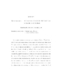## Autonomous Stochastic Perturbations of Hamiltonian Systems##### Files
umi-umd-1663.pdf(587.92 KB)
We consider autonomous stochastic perturbations $\dot X^{\varepsilon}(t)=\skewgrad H(X^{\varepsilon}(t))+\varepsilon b(X^{\varepsilon}(t))$ of Hamiltonian systems of one degree of freedom whose Hamiltonian $H$ is quadratic in a neighborhood of the only saddle point of $H$. Assume that $b=b_1+\xi b_2$ for some random fields $b_i$, $i=1,\,2$, and $\xi$ is a random variable, and that ${\rm div}b_i&lt;0$ and $\xi&gt;0$ with probability $1$. Also assume that $H$ has only one saddle point and two minima. To consider the effects of the perturbations, we consider the graph $\Gamma$ homeomorphic to the space of connected components of the level curves of $H$ and the processes $Y^{\varepsilon}_t$ on $\Gamma$ which represent the slow component of the motion of the perturbed system. We show that as $\varepsilon\rightarrow 0$, the processes $Y^{\varepsilon}_t$ tend to a certain stochastic process $Y_t$ on $\Gamma$ which can be determined inside the edges by a version of the averaging principle and branches at the interior vertex into adjacent edges with certain probabilities which can be calculated by $H$ and the perturbation $b$. Also our result can be used to regularize some deterministic perturbations, partially coinciding with the results obtained by Brin and Freidlin in a earlier work.